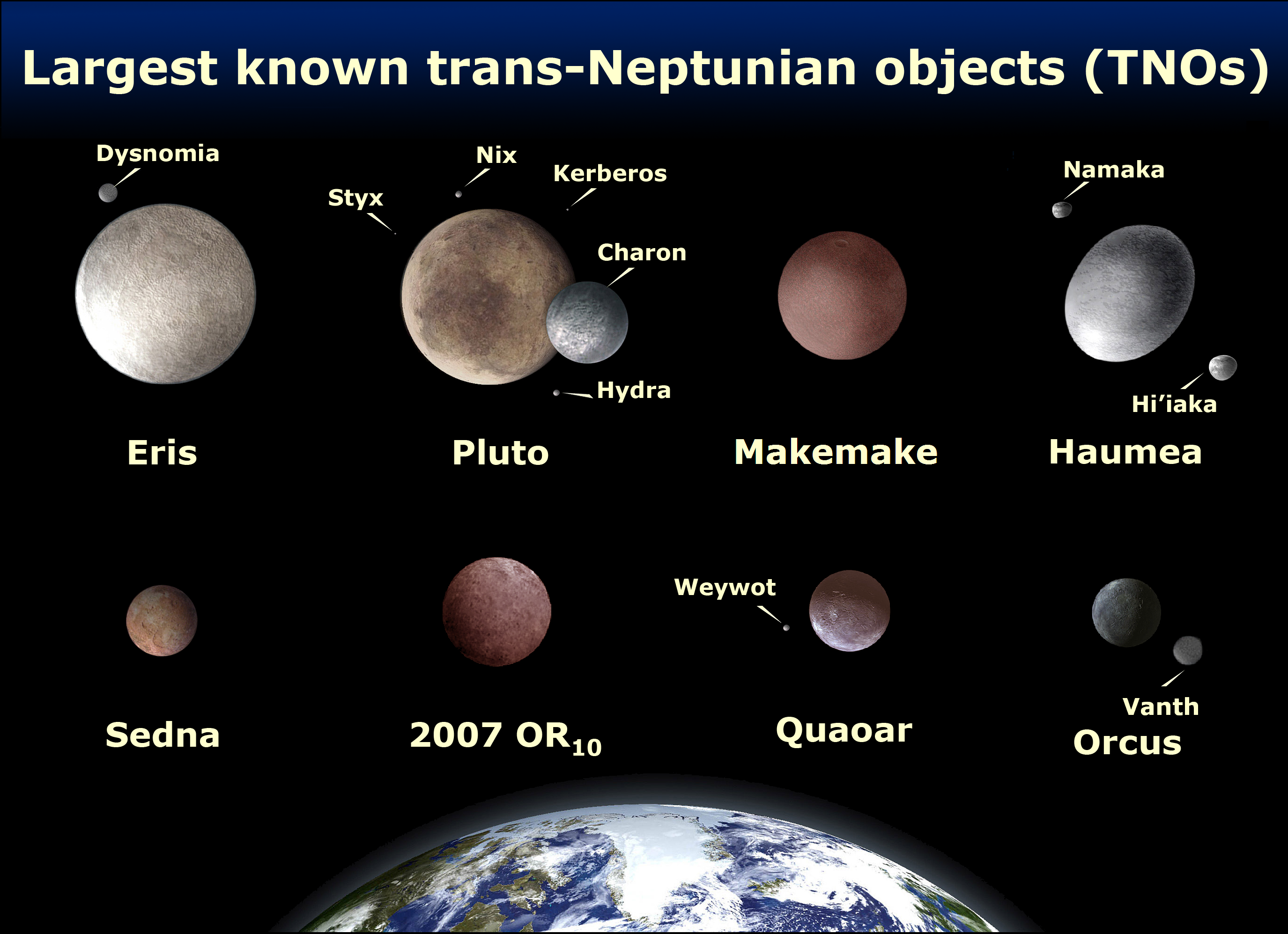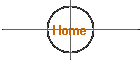### Classification of the Planets and Their Physical Properties

The planets of the modern solar system are grouped into several different and sometimes overlapping classifications, as illustrated here: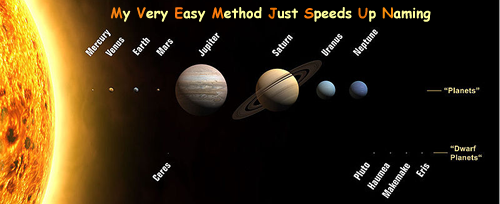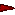The planets inside the orbit of the earth are called the Inferior Planets: Mercury and Venus.The planets outside the orbit of the earth are called the Superior Planets: Mars, Jupiter, Saturn, Uranus, Neptune, and Pluto.The planets inside the asteroid belt are termed the Inner Planets (or the Terrestrial Planets): Mercury, Venus, Earth, and Mars.The planets outside the asteroid belt are termed the Outer Planets: Jupiter, Saturn, Uranus, and Neptune.The planets sharing the gaseous structure of Jupiter are termed the Gas Giant (or Jovian) Planets: Jupiter, Saturn, Uranus, and Neptune.Dwarf Planets

The Nine (8) Planets is an overview of the history, mythology, and current scientific knowledge of each of the planets and moons in our solar system. Each page has text and images, some have sounds and movies, most provide references to additional related information.

### Physical Properties of the Planets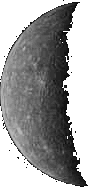MERCURY Distance from the Sun: Mean: 57.9 million km (0.387 astronomical units) Max: 69.7 million km (0.467 a.u.) Min: 45.9 million km (0.306 a.u.) Sidereal period: 87.969 days Synodic period: 115.88 days Rotation period: 58.6461 days Mean orbital velocity: 47.87 km/s Axial inclination: Diameter: 4878 km Surface area: 7.475 x 107 Density, water = 1:5.44 Mass: 3.3 x 1026 g Mass, Earth = 1:0.055 Volume, Earth = 1:0.056 Escape velocity: 4.25 km/s Surface gravity, Earth = 1:0.38 Mean surface temperature: Day +350°C Night -170°C Extremes of surface temperature: Day +427°C Night -183°C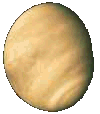VENUS Distance from the Sun: Mean: 108.2 million km (0.723 a.u.) Max: 109 million km (0.728 a.u.) Min: 107.4 million km (0.718 a.u.) Sidereal period: 224.701 days Synodic period: 583.9 days Rotation period: 243.16 days Mean orbital velocity: 35.02 km/s Axial inclination: 178 deg Diameter: 12104 km  Density, water = 1:5.25 Mass: 4.870 x 1027 g Mass, Earth = 1:0.815 Volume, Earth = 1: 0.86 Escape velocity: 10.36 km/s Surface gravity, Earth = 1:0.903 Mean surface temperature: Cloud tops -33°C Surface +480°CMARS Distance from the Sun: Mean: 227.94 million km (1.524 a.u.) Max: 249.1 million km (1.666 a.u.) Min: 206.7 million km (1.381 a.u.) Sidereal period: 686.980 days Synodic period: 779.9 days Rotation period: 24 h 37 m 22.6 s Mean orbital velocity: 24.1 km/s Axial inclination: 23 deg 59 min Diameter: 6794 km  Density, water = 1:3.94 Mass: 6.421 x 1026 g Mass, Earth = 1:0.107 Volume, Earth = 1:0.150 Escape velocity: 5.03 km/s Surface gravity, Earth = 1:0.380 Mean surface temperature: -23°CJUPITER Distance from the Sun: Mean: 778.34 million km (5.203 a.u.) Max: 815.7 million km (5.455 a.u.) Min: 740.9 million km (4.951 a.u.) Sidereal period: 11.68 years Synodic period: 398.9 days Rotation period: 9 h 50 m 30 s Mean orbital velocity: 13.06 km/s Axial inclination: 3 deg 04 min Diameter: 143884 km  Density, water = 1:1.33 Mass, Earth = 1:317.89 Volume, Earth = 1:1318.7 Escape velocity: 60.22 km/s Surface gravity, Earth = 1:2.64 Mean surface temperature: -150°C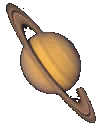SATURN Distance from the Sun: Mean: 1427 million km (9.539 a.u.) Max: 1507 million km (10.069 a.u.) Min: 1347 million km (9.008 a.u.) Sidereal period: 29.46 years Mean synodic period: 378.1 days Rotation period: 10 h 13 m 59 s Mean orbital velocity: 9.6 km/s Axial inclination: 26 deg 44 min Diameter: 120536 km  Density, water = 1:0.71 Mass, Earth = 1:95.17 Volume, Earth = 1:744 Escape velocity: 32.26 km/s Surface gravity, Earth = 1:1.16 Mean surface temperature: -180°C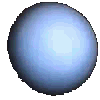URANUS Distance from the Sun: Mean: 2869.6 million km (19.181 a.u.) Max: 3004 million km (20.088 a.u.) Min: 2735 million km (18.275 a.u.) Sidereal period: 84.01 years Synodic period: 369.7 days Rotation period: 17.24 hours Mean orbital velocity: 6.90 km/s Axial inclination: 98 deg Diameter: 51118 km  Density, water = 1:1.27 Mass, Earth = 1:14.6 Volume, Earth = 1:67 Escape velocity: 22.5 km/s Surface gravity, Earth = 1:1.17 Mean surface temperature: -214°C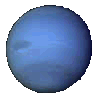NEPTUNE Distance from the Sun: Mean: 4496.7 million km (30.058 a.u.) Max: 4537 million km (30.316 a.u.) Min: 4456 million km (29.800 a.u.) Sidereal period: 164.8 years Synodic period: 367.5 days Rotation period: 16.1 hours Mean orbital velocity: 5.42 km/s Axial inclination: 28 deg 48 min Diameter: 50538 km  Density, water = 1:2.0643 Mass, Earth = 1:17.2 Volume, Earth = 1:57 Escape velocity: 23.9 km/s Surface gravity, Earth = 1:1.2 Mean surface temperature: -220°C Pluto: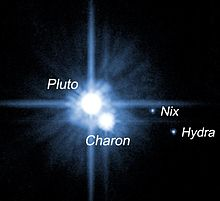PLUTO (Dwarf Planet; Trans-Neptunian Object; Plutoid; Kuiper Belt Object; Plutino) Distance from the Sun: Mean: 5900 million km (39.5 a.u.) Max: 7375 million km (49.28 a.u.) Min: 4425 million km (29.65 a.u.) Sidereal period: 247.7 years Synodic period: 366.7 days Rotation period: 6 d 9 h 17 m 36 s Mean orbital velocity: 4.7 km/s Axial inclination: 122 deg .46 Diameter: 2324 km  Density, water = 1:2.03 Mass, Earth = 1:0.0022 Escape velocity: 1.18 km/s Surface gravity, Earth = 1:0.06 Mean surface temperature: -230°C Moons: Charon, Nix, Hydra, Styx, Kerberos